# IB DP Physics: SL复习笔记5.2.4 I-V Characteristics

### Ohm's Law

• Ohm's law states that:

For a conductor at a constant temperature, the current through it is proportional to the potential difference across it

• An electrical component obeys Ohm’s law if its graph of current against potential difference is a straight line through the origin
• A fixed resistor obeys Ohm’s law - i.e. it is an ohmic component
• A filament lamp does not obey Ohm’s law - it is a non-ohmic componentThe current-voltage graph for a fixed resistor is a straight line through the origin. The fixed resistor is an ohmic component

• The resistance of an ohmic component can be calculated from the gradient of its current-voltage graph
• Since resistance is R = V/I

#### Worked Example

The current flowing through a component varies with the potential difference V across it as shown.Which graph best represents how the resistance R varies with V?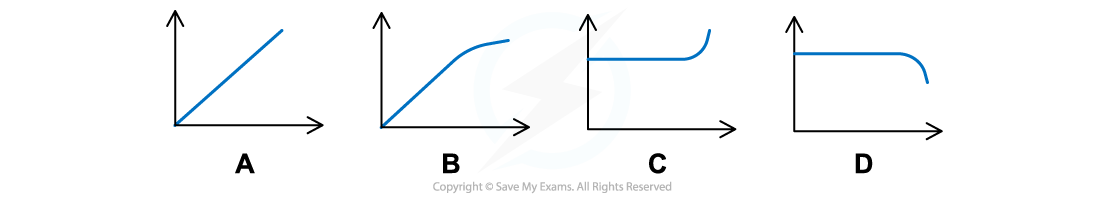Step 1: Write down the equation for the resistance R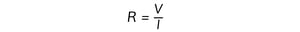Step 3: Identify the gradient of different sections of the graph and use it to deduce what happens to the resistance

• The first straight section of the graph has a constant gradient
• So the resistance remains constant
• The second section is curved and the steepness of the line increases, so the gradient increases
• So the resistance decreases

Step 4: Identify the correct graph out of the four proposed

• Constant resistance is indicated by a straight horizontal line
• So either C or D are correct
• Decreasing resistance is indicated by a line curving downwards
• So only D is correct

#### Exam Tip

When solving problems about Ohm's law you will often deal with graphs. You need to be confident identifying and calculating their gradients.

• In maths, the gradient is the slope of the graph (i.e. rise/run)
• The graphs below show a summary of how the slope of the graph represents the gradient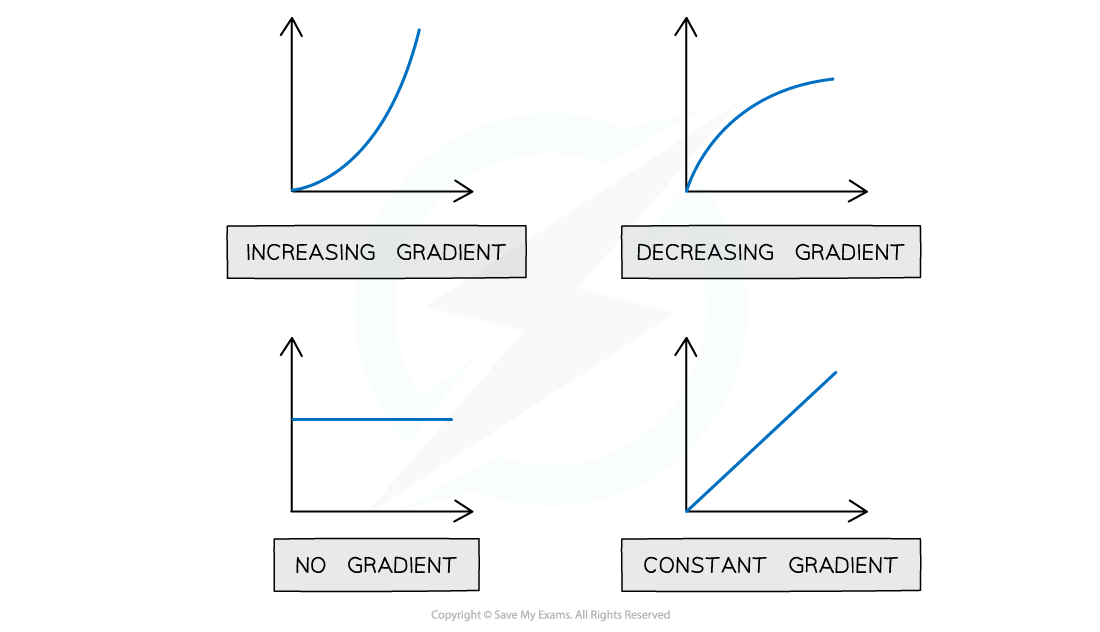### I-V Characteristics

• The I-V characteristics of a circuit component is a graph of the current flowing through the component plotted against the voltage across it

#### Obtaining I-V Characteristics Experimentally

• The relation between potential difference across an electrical component (e.g. a fixed resistor) and current can be investigated through a circuit such as the one below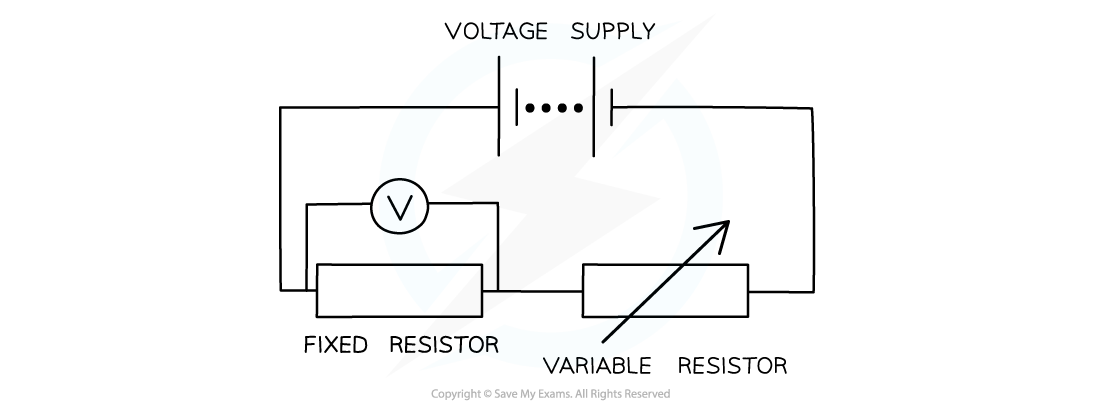Circuit for plotting graphs of current against voltage. The component being investigated here is a fixed resistor

• By adjusting the resistance on the variable resistor:
• The current in the circuit will change
• For each value of the current I, the potential difference V can be recorded
• A graph of current against potential difference can be plotted

#### I-V Graphs of Some Circuit Components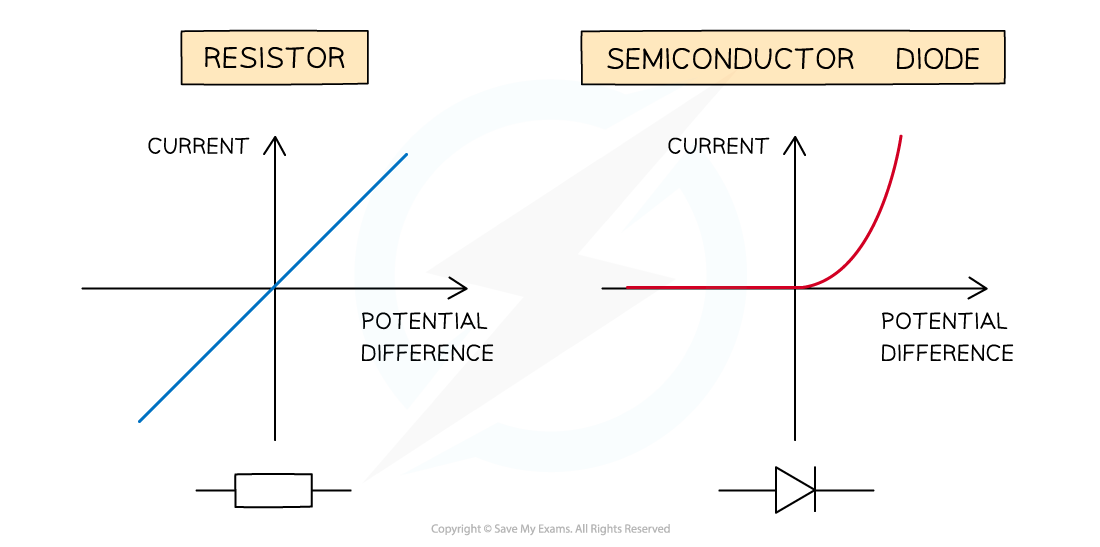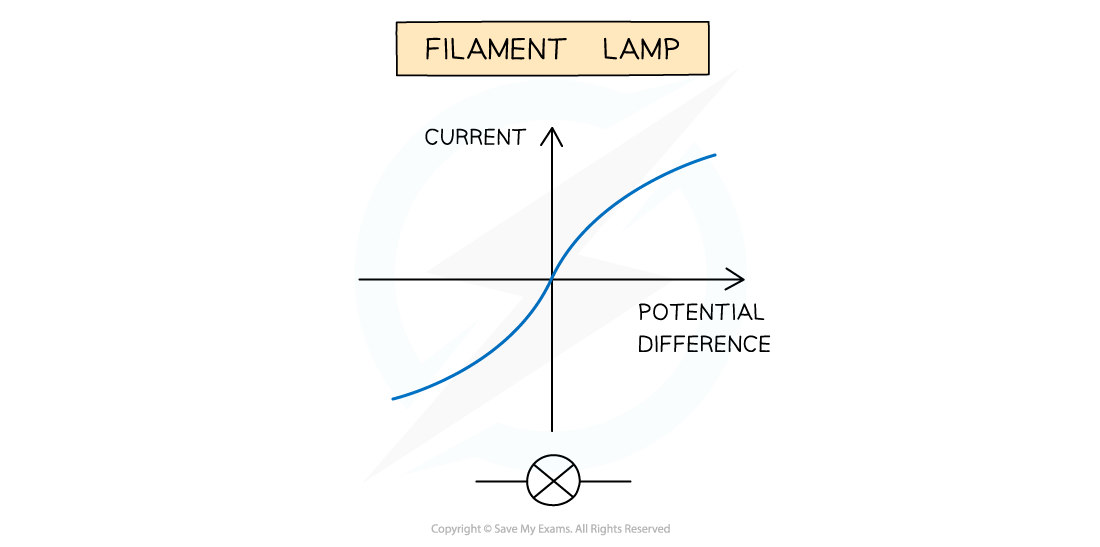I–V characteristics for an ohmic conductor (e.g. resistor), semiconductor diode and filament lamp

Ohmic Conductor

• Current is directly proportional to potential difference
• The I-V graph is a straight line through the origin

Semiconductor Diode

• When the current is in the direction of the arrowhead symbol, the diode is said to be forward biased
• There is a sharp increase in current
• This is shown on the right side of the graph
• When the diode is switched around, it does not conduct and it is said to be reverse biased
• The current through the diode is zero
• This is shown on the left side of the graph
• The diode is a non-ohmic component
• Its I-V graph is not a straight line through the origin

Filament Lamp

• For very small voltages, the filament lamp behaves as an ohmic component
• The middle section of the graph (around zero voltage) is straight and passes through the origin
• As voltage increases:
• More current flows through the filament lamp and the temperature of the filament in the lamp increases
• The higher the temperature of the filament, the higher its resistance
• Since resistance opposes current, the current flows through the filament at a slower rate
• This is shown by the curved section of the graph
• For slightly higher voltages, the filament lamp is non-ohmic
• The I-V graph is a curve with decreasing gradient

#### Exam Tip

Make sure you're confident in drawing the I–V characteristics for different components, as you may be asked to sketch these from memory or to identify those given in exam questions